# Stability theorems in algebraic K-theory

(diff) ← Older revision | Latest revision (diff) | Newer revision → (diff)

Assertions on the invariance of the groups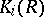or their subgroups, given certain special extensions of the ground ring(see Algebraic-theory).

The following are the best-known stability theorems. Letbe a regular ring (cf. Regular ring (in commutative algebra)) and let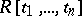be the ring of polynomials in the variables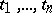over. The stability theorem for Whitehead groups under the transfer fromto, , states that the natural homomorphism imbeddingininduces an isomorphism between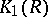and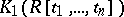(cf. also Whitehead group).

In the case of a skew-fieldthat is finite-dimensional over its centre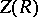, one can define a reduced-norm homomorphismof the multiplicative group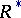ofinto the multiplicative group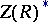of its centre. The kernel of this homomorphism, usually written as, determines the reduced Whitehead group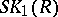of: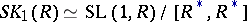(see Special linear group), which is a subgroup in. If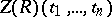is the field of rational functions inover, then the algebrais a skew-field, and the natural imbeddingofininduces a homomorphism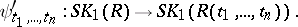The stability theorem for reduced Whitehead groups states that the homomorphism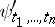is bijective (, see also ). Similar statements are also true in unitary and spinor algebraic-theories , .

Theorems on stabilization for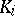-functors under the transfer from the stable objectsto unstable ones are also called stability theorems (see ).

How to Cite This Entry:
Stability theorems in algebraic K-theory. Encyclopedia of Mathematics. URL: http://encyclopediaofmath.org/index.php?title=Stability_theorems_in_algebraic_K-theory&oldid=12794
This article was adapted from an original article by V.I. Yanchevskii (originator), which appeared in Encyclopedia of Mathematics - ISBN 1402006098. See original article